## Methods and code for the manuscript quantifying land fragmentation metrics for cattle enterprises in Northern Ireland

This is an R Markdown document which should make the analytical steps clear and repeatable. In lieu of providing the original spatial data (due to GDPR concerns), we present definitions, summary data and visual distributions of the research data.

### 1. Metadata on herd type and herd size

There are 19008 Herd IDs and 17744 Business IDs across the three study years.

``````dat1 %>% group_by(year) %>%
summarise(
n.herds = n_distinct(HerdId)
``````## # A tibble: 3 x 3
##   <fct>   <int>        <int>
## 1 2015    19008        17744
## 2 2016    19008        17744
## 3 2017    19008        17744``````

Whilst business IDs can be associated with more than one herd, in the vast majority of cases, there was a 1:1 mapping between Business IDs and Herd IDs; out of 17744 businesses, 1192 were associated with more than one Herd ID (i.e. 6.72%)

``````dat1 %>% group_by(BusinessId) %>%
summarise(
n.herds.per.bus.id = n_distinct(HerdId)) %>%
filter(n.herds.per.bus.id > 1) %>%
``````## # A tibble: 1 x 1
##   n.bus.multip.herds
##                <int>
## 1               1192``````

Whilst herds could change production type year on year, The number of different herd types remained broadly consistent across the three years of the study

``````ggplot(dat1, aes(x = year, fill = HerdType)) +
geom_bar(stat="count") +
scale_fill_viridis(discrete = TRUE) +
labs(x = "Year", y = "Number of herds", fill = "Herd Type") +
theme_bw() ``````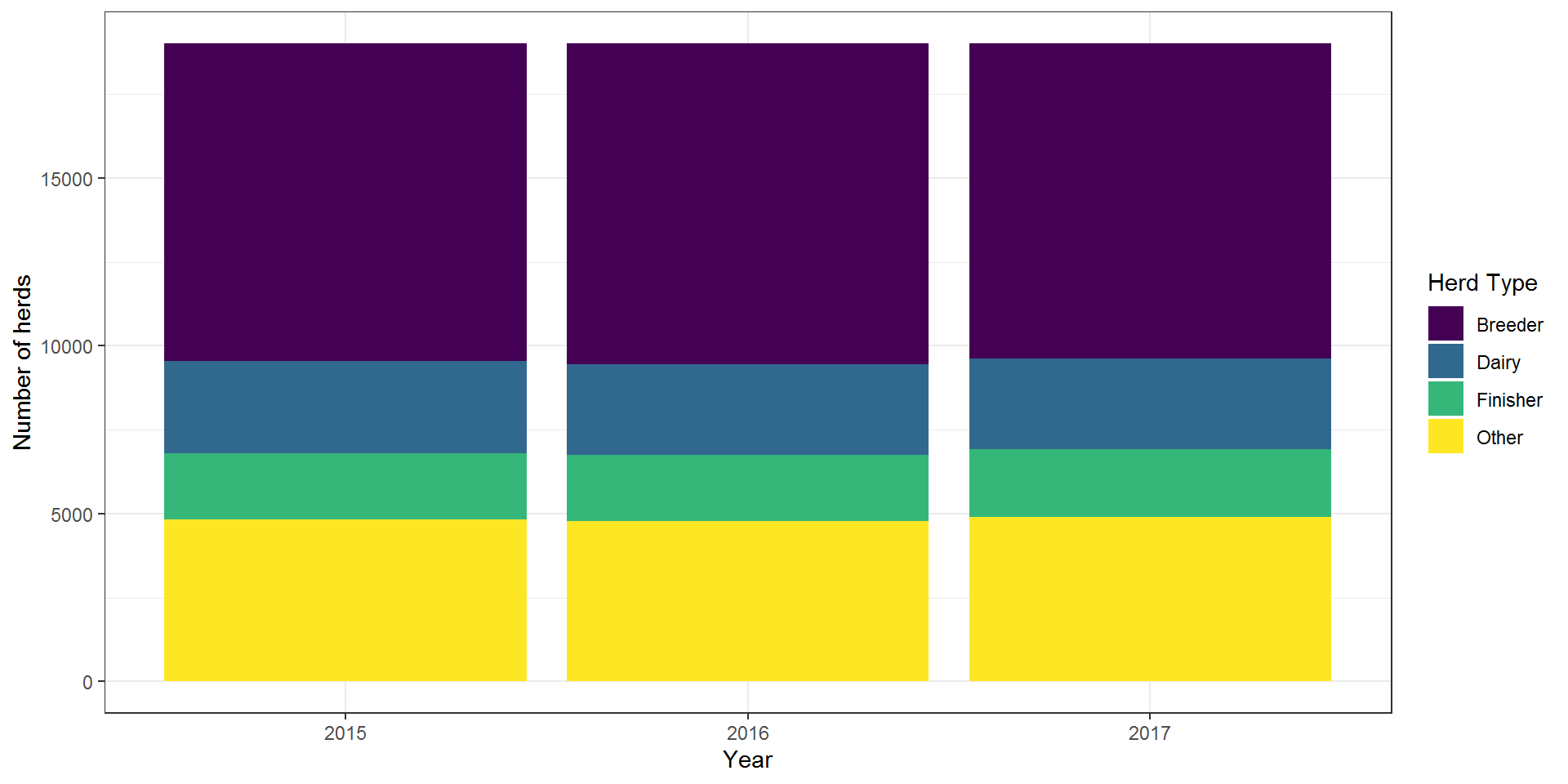There is no notable difference in herd types between years.

``````orig_table <- table(dat1\$HerdType, dat1\$year)
round(prop.table(orig_table, margin = 2),2)``````
``````##
##            2015 2016 2017
##   Breeder  0.50 0.50 0.49
##   Dairy    0.14 0.14 0.14
##   Finisher 0.10 0.10 0.11
##   Other    0.25 0.25 0.26``````
``chisq.test(orig_table)``
``````##
##  Pearson's Chi-squared test
##
## data:  orig_table
## X-squared = 5.3394, df = 6, p-value = 0.5011``````

Median herd size varied with production type and also remained broadly consistent across the three years of the study, within herd types.

``````dat1 %>% group_by(HerdType) %>%
summarise(
"Median herd size"
, q1 = quantile(median_herd_size)
, median = median(median_herd_size)
, q2. = quantile(median_herd_size)
, max = max(median_herd_size))``````
``````## # A tibble: 4 x 6
##   HerdType `"Median herd size"`    q1 median   q2.   max
##   <chr>    <chr>                <dbl>  <dbl> <dbl> <dbl>
## 1 Breeder  Median herd size        17   30      54  904.
## 2 Dairy    Median herd size        96  171     277 1836
## 3 Finisher Median herd size        21   45.5    97 1492.
## 4 Other    Median herd size        15   38      84 1208``````
``````ggplot(dat1, aes(x = HerdType, y = median_herd_size, fill = HerdType)) +
geom_boxplot() +
scale_fill_viridis(discrete = TRUE) +
labs(x = "Herd Type", y = "Median herd size", fill = "Herd Type") +
theme_bw() +
facet_wrap(~year)``````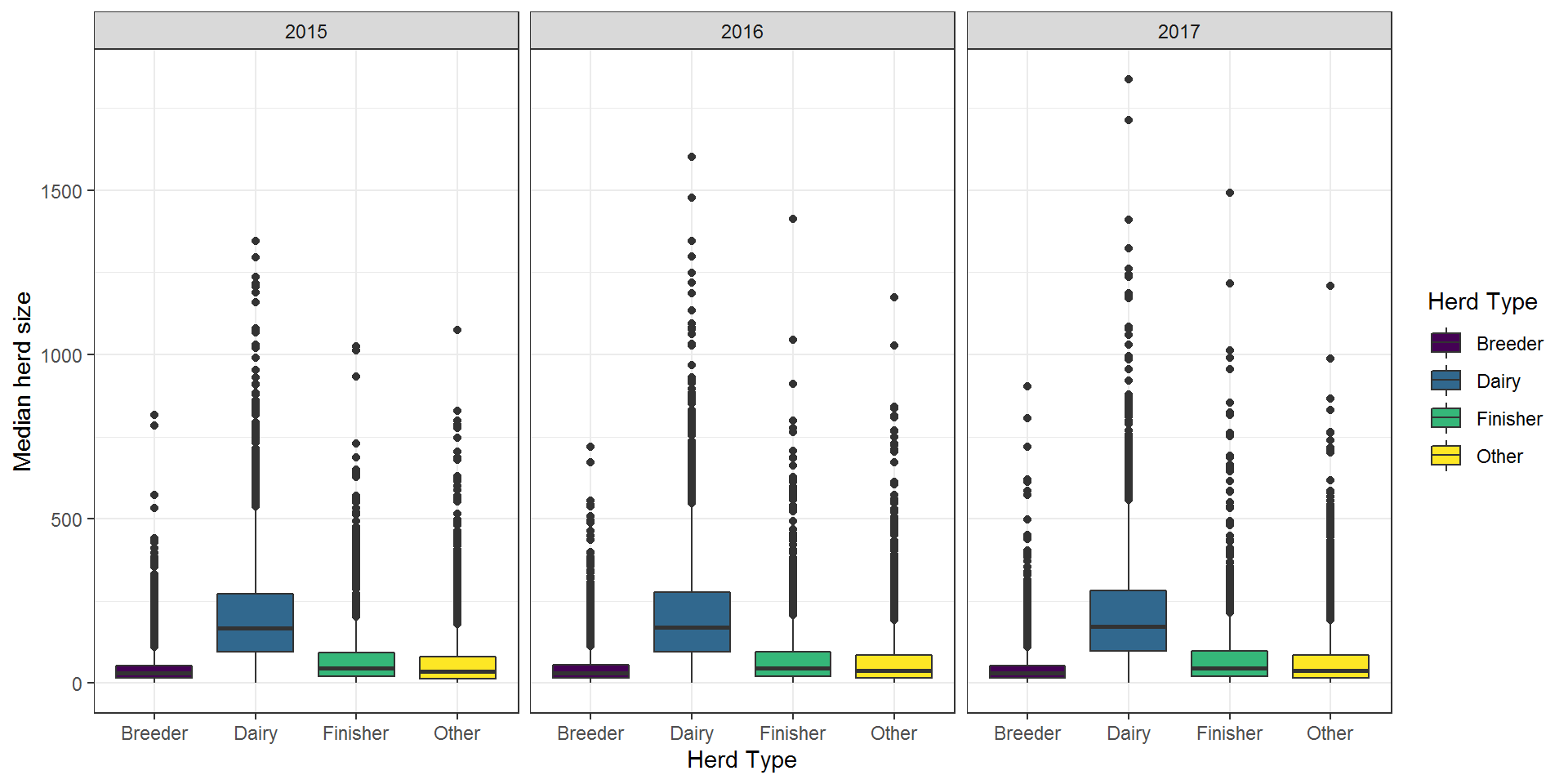### 2. Research data

#### 2.1. Fragmentation metrics

##### n_fields - Number of land parcels

The number of individual land parcels associated with each farm.

``````dat1 %>% group_by(year) %>%
summarise(
"Number of land parcels"
, q1= quantile(n_fields)
, median = median(n_fields)
, q3 = quantile(n_fields)
, max = max(n_fields))``````
``````## # A tibble: 3 x 6
##   year  `"Number of land parcels"`    q1 median    q3   max
##   <fct> <chr>                      <dbl>  <dbl> <dbl> <int>
## 1 2015  Number of land parcels        14     24    39   413
## 2 2016  Number of land parcels        14     24    39   423
## 3 2017  Number of land parcels        14     24    39   444``````
``````ggplot(dat1, aes(x = as.factor(year), y = n_fields)) +
geom_violin() +
geom_boxplot(width=0.1) +
labs(x = "Year", y = "Number of land parcels") +
theme_bw() ``````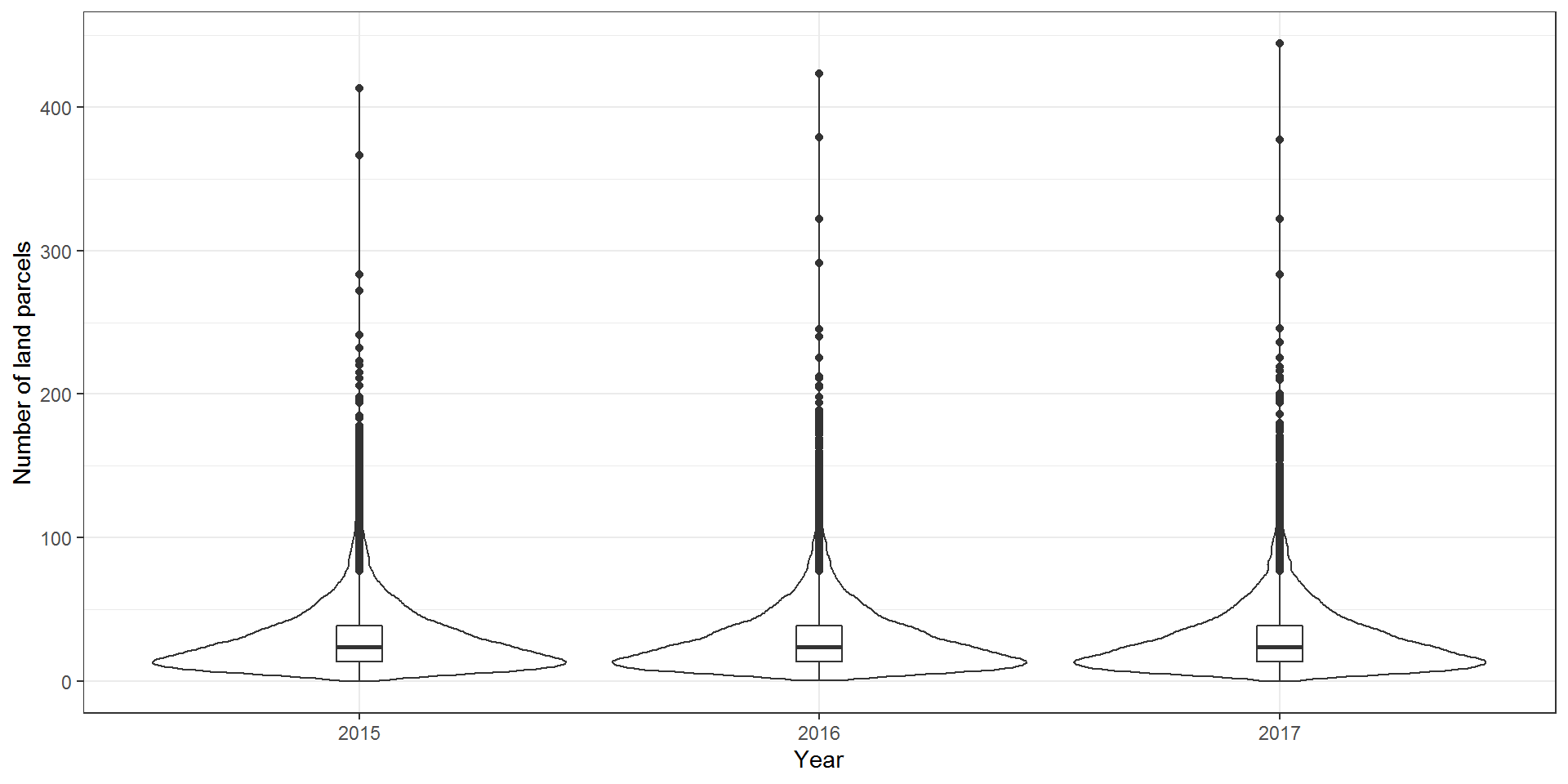No variation the number of land parcels at the farm level between years.

``````tst2 <- dat1 %>% select(HerdType, year, n_fields)
anova(glm(n_fields ~ year, data = tst2), test = "F")``````
``````## Analysis of Deviance Table
##
##
## Response: n_fields
##
## Terms added sequentially (first to last)
##
##
##      Df Deviance Resid. Df Resid. Dev      F Pr(>F)
## NULL                 57023   36362407
## year  2   268.73     57021   36362138 0.2107   0.81``````
##### n_fragments - Number of fragments

The number of land parcel "units" associated with each farm.

``````dat1 %>% group_by(year) %>%
summarise(
"Number of fragments"
, q1 = quantile(n_fragments)
, median = median(n_fragments)
, q3 = quantile(n_fragments)
, max = max(n_fragments))``````
``````## # A tibble: 3 x 6
##   year  `"Number of fragments"`    q1 median    q3   max
##   <fct> <chr>                   <dbl>  <dbl> <dbl> <int>
## 1 2015  Number of fragments         4      6    10    80
## 2 2016  Number of fragments         4      6    10    81
## 3 2017  Number of fragments         4      6    10    80``````
``````ggplot(dat1, aes(x = as.factor(year), y = n_fragments)) +
geom_violin() +
geom_boxplot(width=0.1) +
labs(x = "Year", y = "Number of fragments") +
theme_bw() ``````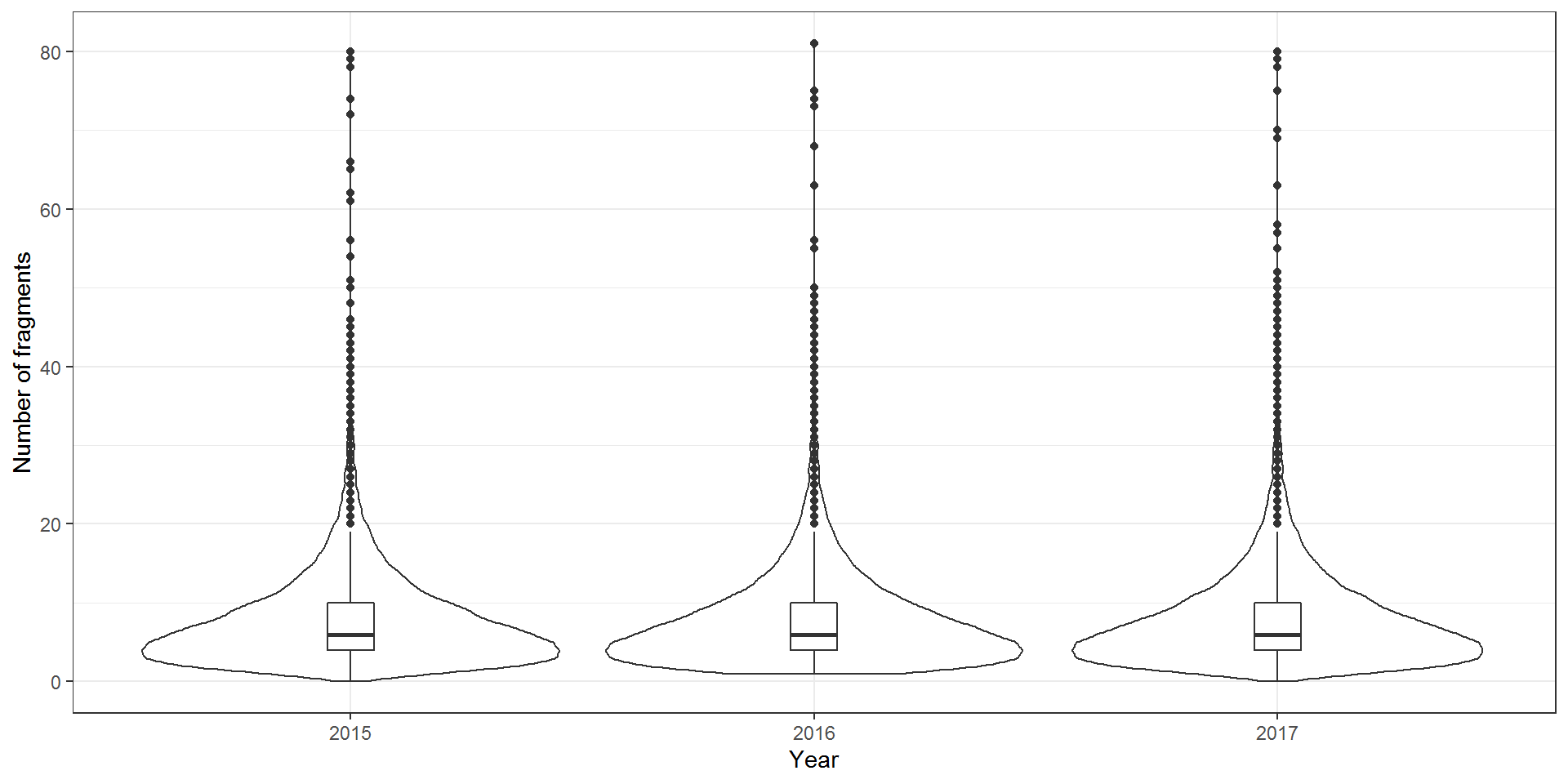No variation the number of fragments at the farm level between years.

``````tst3 <- dat1 %>% select(HerdType, year, n_fragments)
anova(glm(n_fragments ~ year, data = tst3), test = "F")``````
``````## Analysis of Deviance Table
##
##
## Response: n_fragments
##
## Terms added sequentially (first to last)
##
##
##      Df Deviance Resid. Df Resid. Dev      F Pr(>F)
## NULL                 57023    2194821
## year  2   159.74     57021    2194661 2.0751 0.1256``````
##### n_fragments_5m - Number of fragments (5m buffer applied)

The number of land parcel "units" associated with each farm, after the application of a 5m buffer.

``````dat1 %>% group_by(year) %>%
summarise(
"Number of fragments (5m buffer)"
, q1 = quantile(n_fragments_5m)
, median = median(n_fragments_5m)
, q3 = quantile(n_fragments_5m)
, max = max(n_fragments_5m))``````
``````## # A tibble: 3 x 6
##   year  `"Number of fragments (5m buffer)"`    q1 median    q3   max
##   <fct> <chr>                               <dbl>  <dbl> <dbl> <int>
## 1 2015  Number of fragments (5m buffer)         2      3     6    39
## 2 2016  Number of fragments (5m buffer)         2      3     6    47
## 3 2017  Number of fragments (5m buffer)         2      3     6    42``````
``````ggplot(dat1, aes(x = as.factor(year), y = n_fragments_5m)) +
geom_violin() +
geom_boxplot(width=0.1) +
labs(x = "Year", y = "Number of fragments (5m buffer)") +
theme_bw() ``````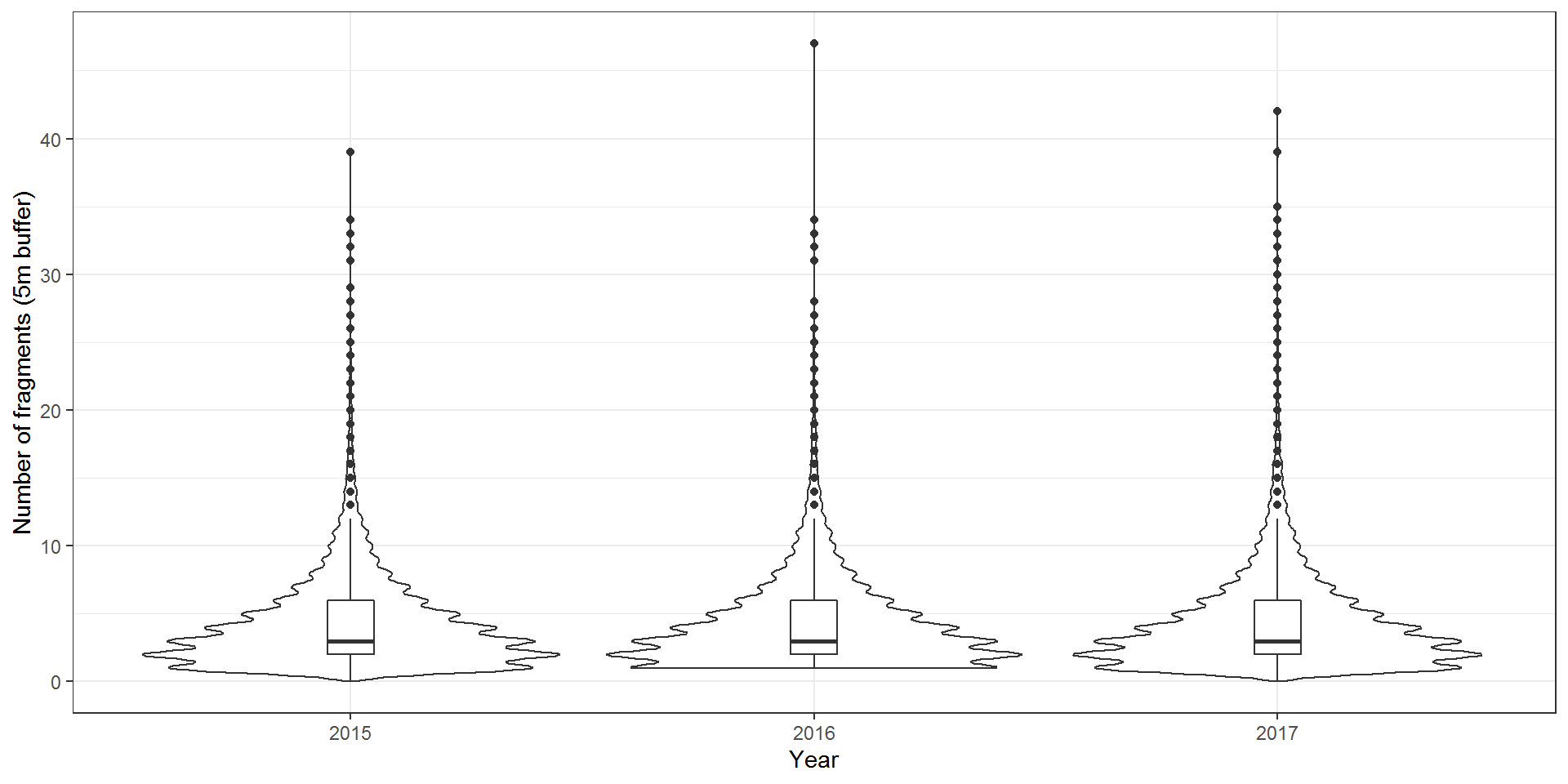No variation the number of fragments at the farm level (5m buffer) between years.

``````tst4 <- dat1 %>% select(HerdType, year, n_fragments_5m)
anova(glm(n_fragments_5m ~ year, data = tst4), test = "F")``````
``````## Analysis of Deviance Table
##
##
## Response: n_fragments_5m
##
## Terms added sequentially (first to last)
##
##
##      Df Deviance Resid. Df Resid. Dev      F Pr(>F)
## NULL                 57023     689708
## year  2   50.729     57021     689657 2.0972 0.1228``````
##### total_farm_area_ha - Farm area

The total farm area in hectares.

``````dat1 %>% group_by(year) %>%
summarise(
"Farm area (ha)"
, q1 = quantile(total_farm_area_ha, na.rm = T)
, median = median(total_farm_area_ha, na.rm = T)
, q3 = quantile(total_farm_area_ha, na.rm = T)
, max = max(total_farm_area_ha, na.rm = T))``````
``````## # A tibble: 3 x 6
##   year  `"Farm area (ha)"`    q1 median    q3   max
##   <fct> <chr>              <dbl>  <dbl> <dbl> <dbl>
## 1 2015  Farm area (ha)      16.5   31.2  59   1403
## 2 2016  Farm area (ha)      16.4   31.1  59.2 3241
## 3 2017  Farm area (ha)      16.4   31.4  59.1 3252.``````
``````ggplot(dat1, aes(x = as.factor(year), y = total_farm_area_ha)) +
geom_violin() +
geom_boxplot(width=0.1) +
labs(x = "Year", y = "Farm area (ha)") +
theme_bw() ``````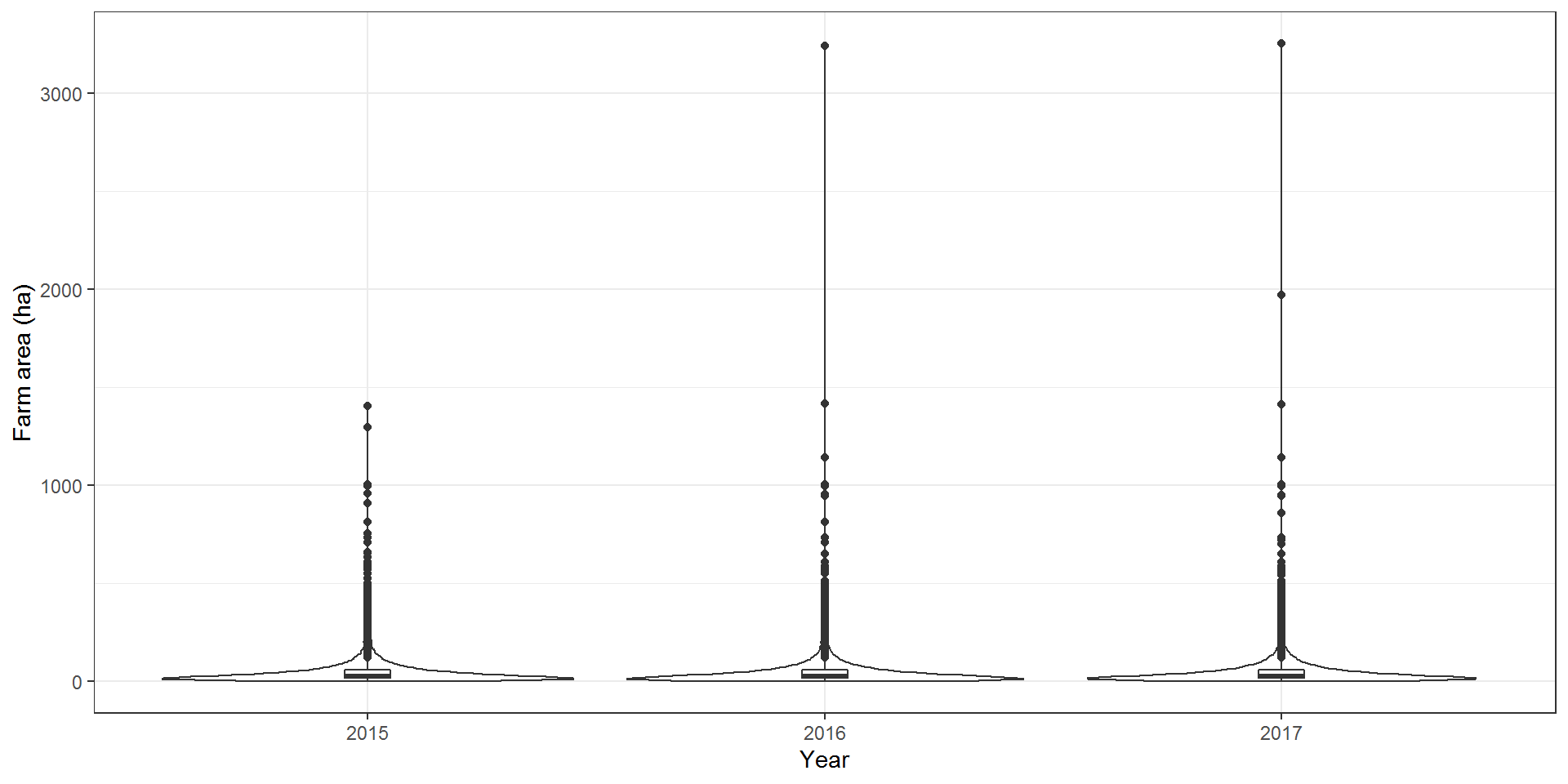No variation in farm area at the farm level between years.

``````tst5 <- dat1 %>% select(HerdType, year, total_farm_area_ha)
anova(glm(total_farm_area_ha ~ year, data = tst5), test = "F")``````
``````## Analysis of Deviance Table
##
##
## Response: total_farm_area_ha
##
## Terms added sequentially (first to last)
##
##
##      Df Deviance Resid. Df Resid. Dev      F Pr(>F)
## NULL                 57023  224573593
## year  2   3521.5     57021  224570071 0.4471 0.6395``````

#### 2.2. Dispersal metrics

##### median_distance_fragments_km - Median distance between fragments

The median distance (in km) between the centroids of all land parcels associated with a cattle farm.

``````dat1 %>% group_by(year) %>%
summarise(
"Median distance between fragments (km)"
, q1 = quantile(median_distance_fragments_km, na.rm = T)
, median = median(median_distance_fragments_km, na.rm = T)
, q3 = quantile(median_distance_fragments_km, na.rm = T)
, max = max(median_distance_fragments_km, na.rm = T))``````
``````## # A tibble: 3 x 6
##   year  `"Median distance between fragments (km)"`    q1 median    q3   max
##   <fct> <chr>                                      <dbl>  <dbl> <dbl> <dbl>
## 1 2015  Median distance between fragments (km)     0.528   1.39  3.04  120.
## 2 2016  Median distance between fragments (km)     0.518   1.36  3.03  120.
## 3 2017  Median distance between fragments (km)     0.518   1.38  3.07  120.``````
``````ggplot(dat1, aes(x = as.factor(year), y = median_distance_fragments_km)) +
geom_violin() +
geom_boxplot(width=0.1) +
labs(x = "Year", y = "Median distance between fragments (km)") +
theme_bw() ``````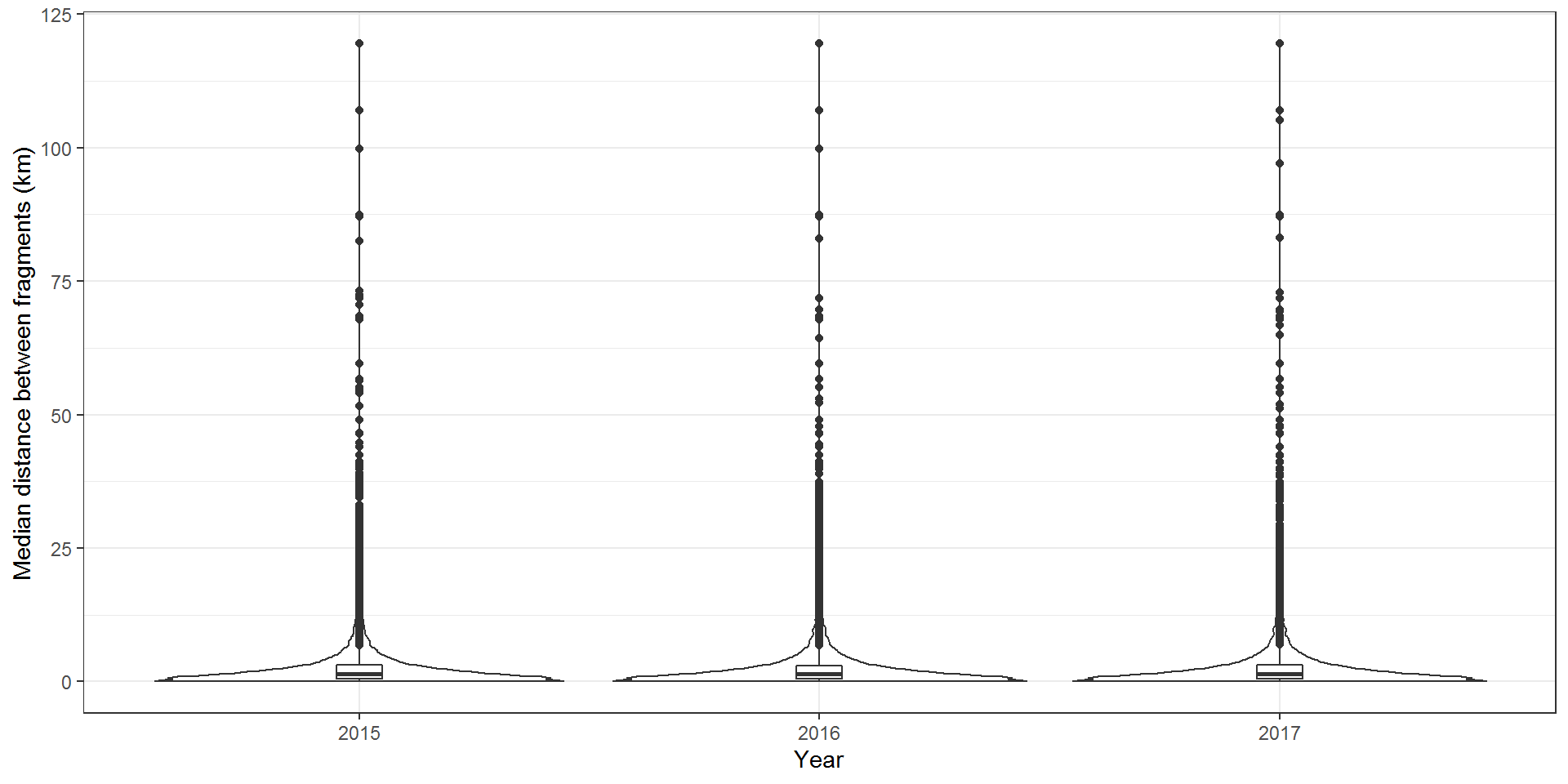No variation in median distance between fragments at the farm level between years.

``````tst6 <- dat1 %>% select(HerdType, year, median_distance_fragments_km)
anova(glm(median_distance_fragments_km ~ year, data = tst6), test = "F")``````
``````## Analysis of Deviance Table
##
##
## Response: median_distance_fragments_km
##
## Terms added sequentially (first to last)
##
##
##      Df Deviance Resid. Df Resid. Dev      F Pr(>F)
## NULL                 57023    1243464
## year  2   22.497     57021    1243442 0.5158  0.597``````
##### max_distance_fragments_km - Maximum distance between fragments

The max distance (in km) between the centroids of the two most distal land parcels associated with a cattle farm.

``````dat1 %>% #group_by(year) %>%
summarise(
"Max distance between fragments (km)"
, q1 = quantile(max_distance_fragments_km, na.rm = T)
, median = median(max_distance_fragments_km, na.rm = T)
, q3 = quantile(max_distance_fragments_km, na.rm = T)
, max = max(max_distance_fragments_km, na.rm = T))``````
``````##   "Max distance between fragments (km)"    q1 median    q3     max
## 1   Max distance between fragments (km) 0.639  2.321 6.088 152.015``````
``````ggplot(dat1, aes(x = as.factor(year), y = max_distance_fragments_km)) +
geom_violin() +
geom_boxplot(width=0.1) +
labs(x = "Year", y = "Max distance between fragments (km)") +
theme_bw() ``````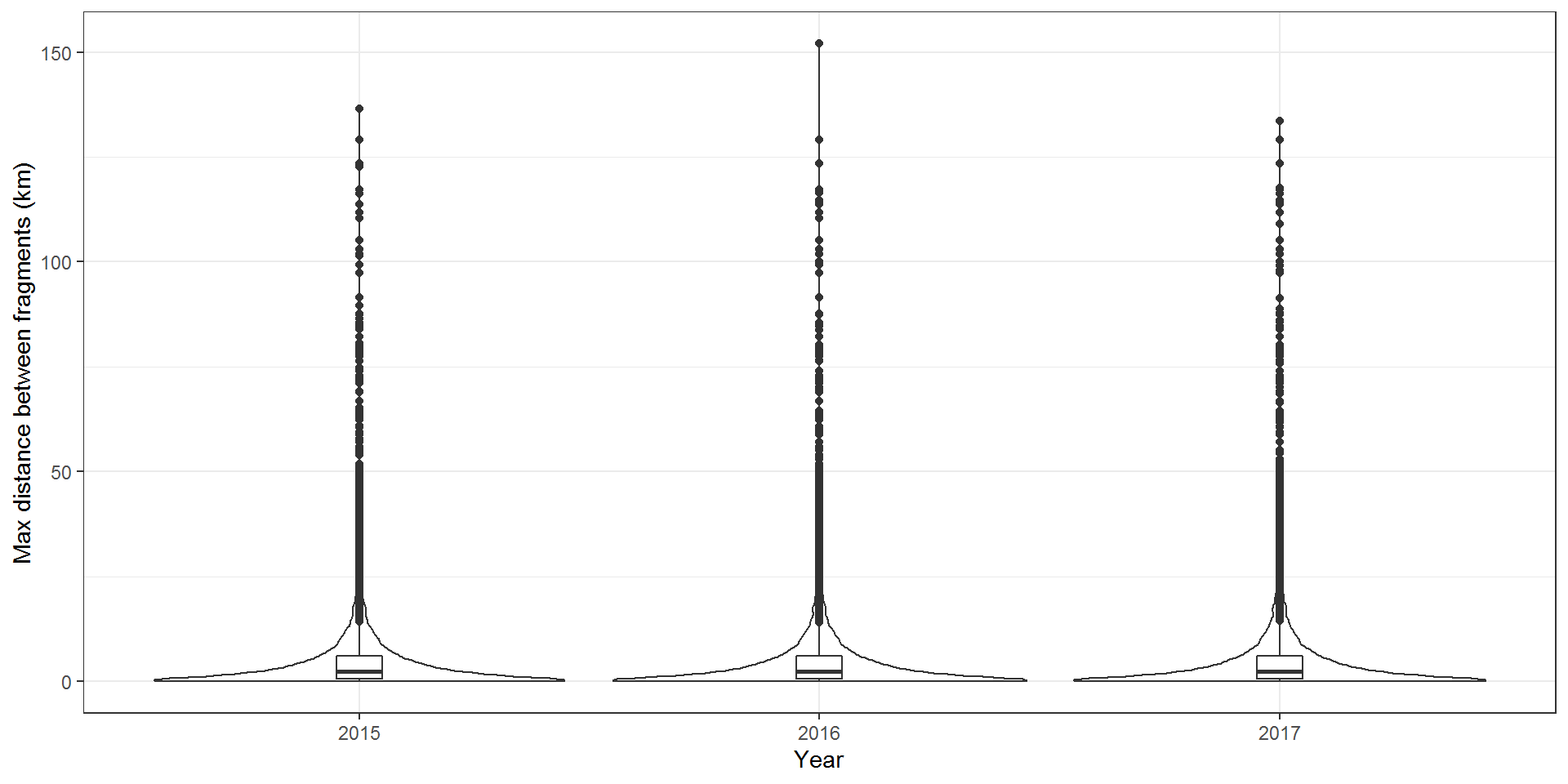No variation in max distance between fragments at the farm level between years.

``````tst7 <- dat1 %>% select(HerdType, year, max_distance_fragments_km)
anova(glm(max_distance_fragments_km ~ year, data = tst7), test = "F")``````
``````## Analysis of Deviance Table
##
##
## Response: max_distance_fragments_km
##
## Terms added sequentially (first to last)
##
##
##      Df Deviance Resid. Df Resid. Dev      F Pr(>F)
## NULL                 57023    4198596
## year  2    142.5     57021    4198453 0.9677   0.38``````
##### Distance between registered homestead and farm centroid, biggest fragement and biggest land parcel

The distances (in km) between the farm homestead (registered address) and the centroid of all land parcels, the centroid of the biggest field and the centroid of the biggest fragment.

``````dat1\$homestead_farmcentroid_dist_km <- round(as.numeric(dat1\$homestead_farmcentroid_dist_km),3)

dat1 %>%
summarise(
"Distance: homestead & farm centroid (km)"
, q1 = quantile(homestead_farmcentroid_dist_km, na.rm = T)
, median = median(homestead_farmcentroid_dist_km, na.rm = T)
, q3 = quantile(homestead_farmcentroid_dist_km, na.rm = T)
, max = max(homestead_farmcentroid_dist_km, na.rm = T))``````
``````##   "Distance: homestead & farm centroid (km)"    q1 median    q3     max
## 1   Distance: homestead & farm centroid (km) 0.273  0.667 1.705 112.756``````
``````dat1 %>%
summarise(
"Distance: homestead & largest parcel (km)"
, q1 = quantile(homestead_field_dist_km, na.rm = T)
, median= median(homestead_field_dist_km, na.rm = T)
, q3 = quantile(homestead_field_dist_km, na.rm = T)
, max = max(homestead_field_dist_km, na.rm = T))``````
``````##   "Distance: homestead & largest parcel (km)"   q1 median    q3    max
## 1   Distance: homestead & largest parcel (km) 0.31  0.686 2.068 127.47``````
``````dat1 %>%
summarise(
"Distance: homestead & largest fragment (km)"
, q1 = quantile(homestead_frag_dist_km, na.rm = T)
, median = median(homestead_frag_dist_km, na.rm = T)
, q3 = quantile(homestead_frag_dist_km, na.rm = T)
, max = max(homestead_frag_dist_km, na.rm = T))``````
``````##   "Distance: homestead & largest fragment (km)"    q1 median      q3    max
## 1   Distance: homestead & largest fragment (km) 0.223 0.4695 1.58325 127.54``````
``````distdf <- dat1 %>% select (HerdId, homestead_farmcentroid_dist_km, homestead_field_dist_km, homestead_frag_dist_km)
distdf\$HerdId <- as.factor(distdf\$HerdId)
names(distdf) <- c("HerdId", "DistanceType", "Distance")``````
``````ggplot(distdf, aes(x = DistanceType, y = Distance)) +
geom_violin() +
geom_boxplot(width=0.1) +
labs(x = "Distance measure", y = "Distance (km)") +
theme_bw() ``````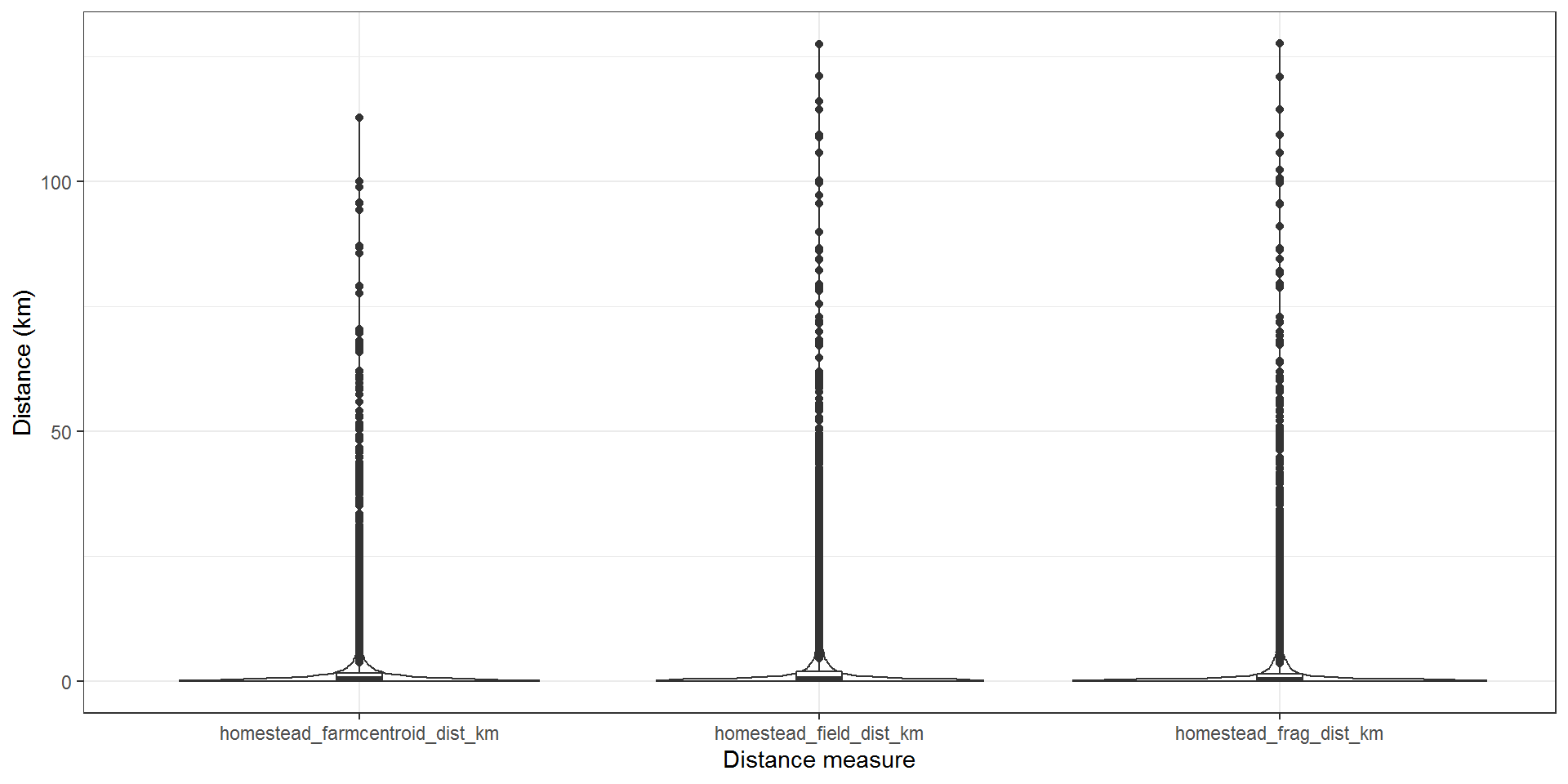#### 2.3. Contact metrics

##### n_touching_cattle_fields_grazing - Number of contiguous internal and external land parcels

The number of external land parcels connected to the index farm, where both internal and external land parcels are suitable for cattle grazing (LCM 4 or 5).

``````dat1 %>% group_by(year) %>%
summarise(
"Number of contiguous grazing fields"
, q1 = quantile(n_touching_cattle_fields_grazing , na.rm = T)
, median = median(n_touching_cattle_fields_grazing, na.rm = T)
, q3 = quantile(n_touching_cattle_fields_grazing, na.rm = T)
, max = max(n_touching_cattle_fields_grazing, na.rm = T))``````
``````## # A tibble: 3 x 6
##   year  `"Number of contiguous grazing fields"`    q1 median    q3   max
##   <fct> <chr>                                   <dbl>  <dbl> <dbl> <int>
## 1 2015  Number of contiguous grazing fields         7     13    21   131
## 2 2016  Number of contiguous grazing fields         7     13    22   167
## 3 2017  Number of contiguous grazing fields         7     12    21   145``````
``````ggplot(dat1, aes(x = as.factor(year), y = n_touching_cattle_fields_grazing)) +
geom_violin() +
geom_boxplot(width=0.1) +
labs(x = "Year", y = "Number of contiguous grazing fields") +
theme_bw() ``````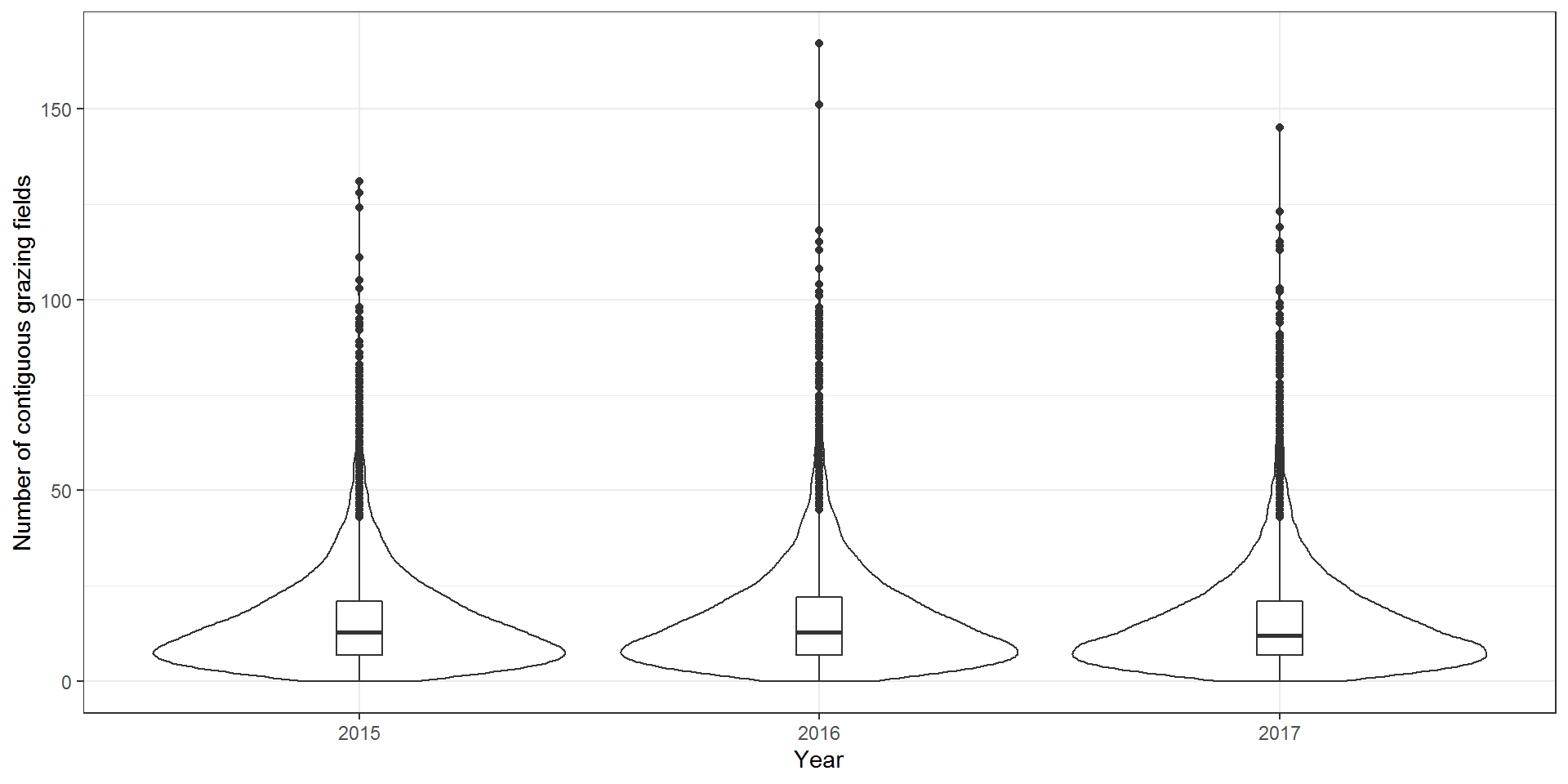Some variation in the number of contiguous grazing fields at the farm level between years, but given the number of tests we have performed, this could be spurious.

``````tst8 <- dat1 %>% select(HerdType, year, n_touching_cattle_fields_grazing)
anova(glm(n_touching_cattle_fields_grazing ~ year, data = tst8), test = "F")``````
``````## Analysis of Deviance Table
##
##
## Response: n_touching_cattle_fields_grazing
##
## Terms added sequentially (first to last)
##
##
##      Df Deviance Resid. Df Resid. Dev      F    Pr(>F)
## NULL                 57023    9757921
## year  2     8233     57021    9749688 24.075 3.537e-11 ***
## ---
## Signif. codes:  0 '***' 0.001 '**' 0.01 '*' 0.05 '.' 0.1 ' ' 1``````
##### total_shared_boundary_grazing_km - The distance (in km) of contact between contiguous internal and external land parcels

The length of the shared perimiter where both internal and external land parcels are suitable for cattle grazing (LCM 4 or 5).

``````dat1 %>% group_by(year) %>%
summarise(
"Length of shared contact boundary (km)"
, q1 = quantile(total_shared_boundary_grazing_km , na.rm = T)
, median = median(total_shared_boundary_grazing_km, na.rm = T)
, q3 = quantile(total_shared_boundary_grazing_km, na.rm = T)
, max = max(total_shared_boundary_grazing_km, na.rm = T))``````
``````## # A tibble: 3 x 6
##   year  `"Length of shared contact boundary (km)"`    q1 median    q3   max
##   <fct> <chr>                                      <dbl>  <dbl> <dbl> <dbl>
## 1 2015  Length of shared contact boundary (km)      1.46   2.81  4.88  44.1
## 2 2016  Length of shared contact boundary (km)      1.54   2.94  5.10  41.1
## 3 2017  Length of shared contact boundary (km)      1.44   2.78  4.85  42.9``````
``````ggplot(dat1, aes(x = as.factor(year), y = total_shared_boundary_grazing_km)) +
geom_violin() +
geom_boxplot(width=0.1) +
labs(x = "Year", y = "Length of shared contact boundary (km)") +
theme_bw() ``````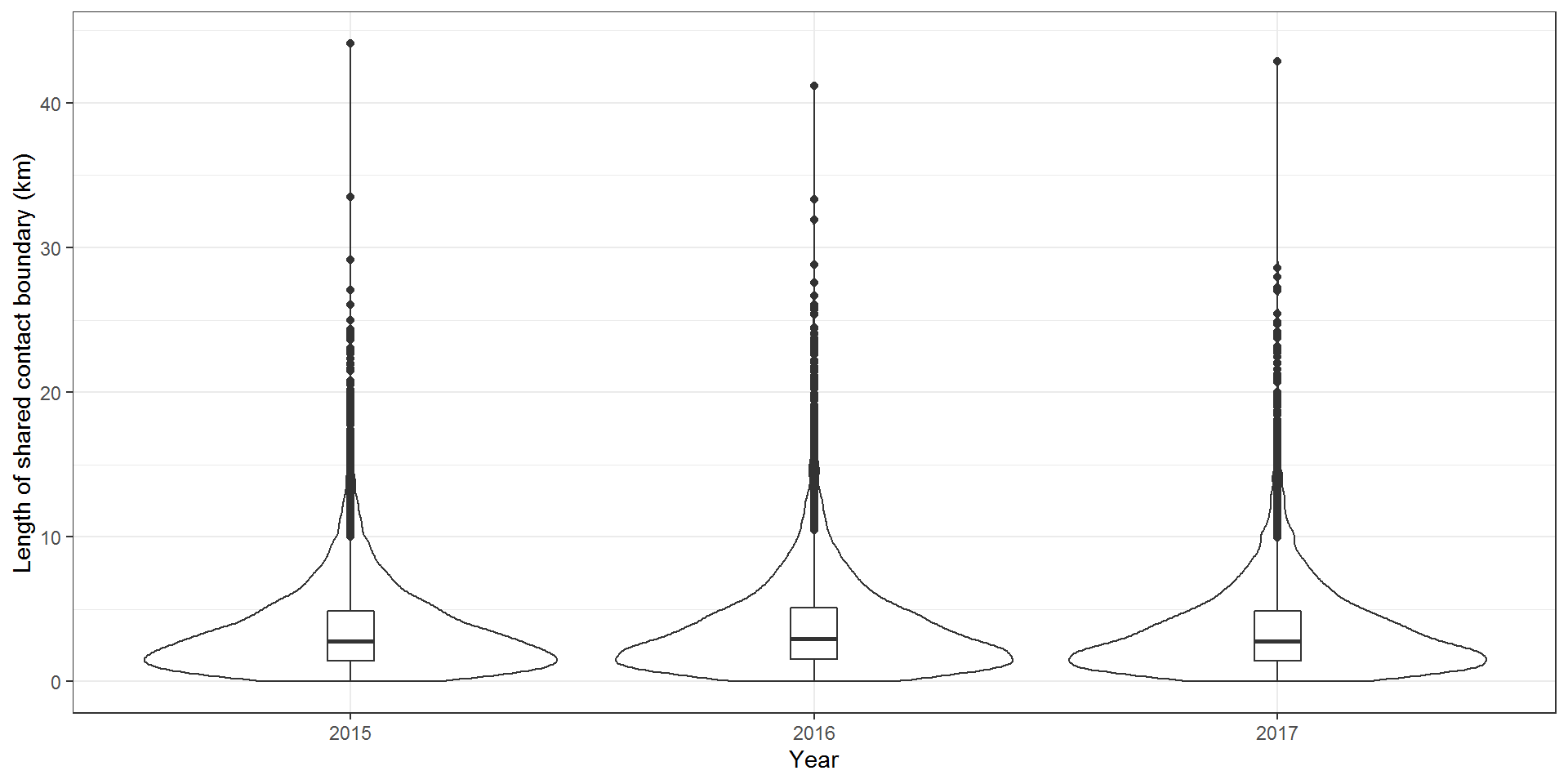Some variation in length of shared contact boundary at the farm level between years, but given the number of tests we have performed, this could be spurious.

``````tst9 <- dat1 %>% select(HerdType, year, total_shared_boundary_grazing_km)
anova(glm(total_shared_boundary_grazing_km ~ year, data = tst9), test = "F")``````
``````## Analysis of Deviance Table
##
##
## Response: total_shared_boundary_grazing_km
##
## Terms added sequentially (first to last)
##
##
##      Df Deviance Resid. Df Resid. Dev      F    Pr(>F)
## NULL                 57023     582419
## year  2   464.15     57021     581954 22.739 1.344e-10 ***
## ---
## Signif. codes:  0 '***' 0.001 '**' 0.01 '*' 0.05 '.' 0.1 ' ' 1``````

#### 2.4. Land classification metrics

##### LCM4_5 - The percentage of land classified as LCM 4 or LCM 5 per farm

The %age area of the farm classified as LCM4 or LCM5 (suitable for grazing)

``````dat1\$LCM4_5 <- round(((dat1\$totLCM4.x + dat1\$totLCM5.x)/dat1\$totSQ)*100,2)

dat1 %>% #group_by(year) %>%
summarise(
"LCM 4 and LCM 5 land on farm (%)"
, q1= quantile(LCM4_5 , na.rm = T)
, median = median(LCM4_5, na.rm = T)
, q3 = quantile(LCM4_5, na.rm = T)
, max. = max(LCM4_5, na.rm = T))``````
``````##   "LCM 4 and LCM 5 land on farm (%)"    q1 median    q3 max.
## 1   LCM 4 and LCM 5 land on farm (%) 70.01   87.8 96.17  100``````
``````ggplot(dat1, aes(x = as.factor(year), y = LCM4_5)) +
geom_violin() +
geom_boxplot(width=0.1) +
labs(x = "Year", y = "LCM 4 and LCM 5 land on farm (%)") +
theme_bw() ``````
``## Warning: Removed 3 rows containing non-finite values (stat_ydensity).``
``## Warning: Removed 3 rows containing non-finite values (stat_boxplot).``